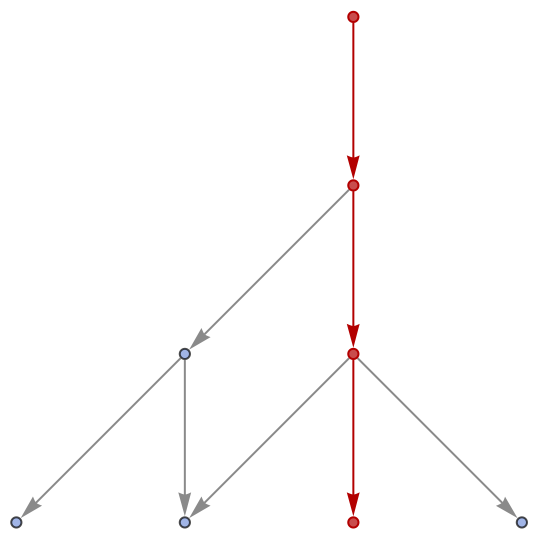#Function Repository Resource:

# FindWolframModelProof

Try to find a proof of equivalence between hypergraphs in a given multiway Wolfram model system

Contributed by: Jonathan Gorard
 ResourceFunction["FindWolframModelProof"][thm,axms] tries to find a proof of the hypergraph equivalence theorem thm using the multiway Wolfram model system axioms axms.

## Details and Options

If ResourceFunction["FindWolframModelProof"][thm,axms] succeeds in deriving the theorem thm from the axioms axms, then it returns a ProofObject expression. If it succeeds in showing that the theorem cannot be derived from the axioms, it returns a Failure object. Otherwise, it may not terminate unless time constrained.
ResourceFunction["FindWolframModelProof"] accepts both individual axioms and lists of axioms, and likewise for theorems.
ResourceFunction["FindWolframModelProof"] has the following options:
 how much time to allow "DirectedHyperedges" whether to treat hyperedges as being ordered (directed)
If ResourceFunction["FindWolframModelProof"] exceeds the specified time constraint, it returns a Failure object.
ResourceFunction["FindWolframModelProof"][thm,axms] uses the Knuth–Bendix completion algorithm to prove that the theorem thm follows from the axioms axms.

## Examples

### Basic Examples (3)

Prove an elementary theorem regarding the equivalence of two hypergraphs in a simple multiway Wolfram model system:

 In:=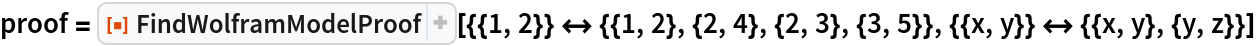Out=Show the abstract proof network, with tooltips showing the intermediate expressions:

 In:=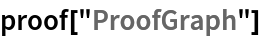Out=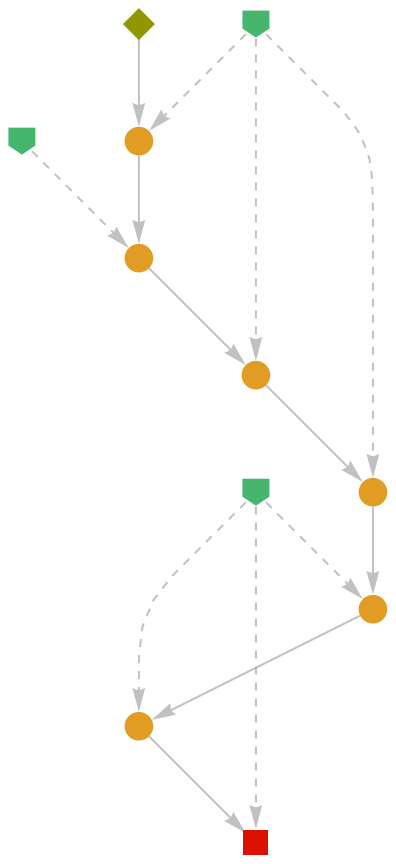Show the complete list of proof steps as a Dataset object:

 In:=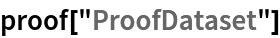Out=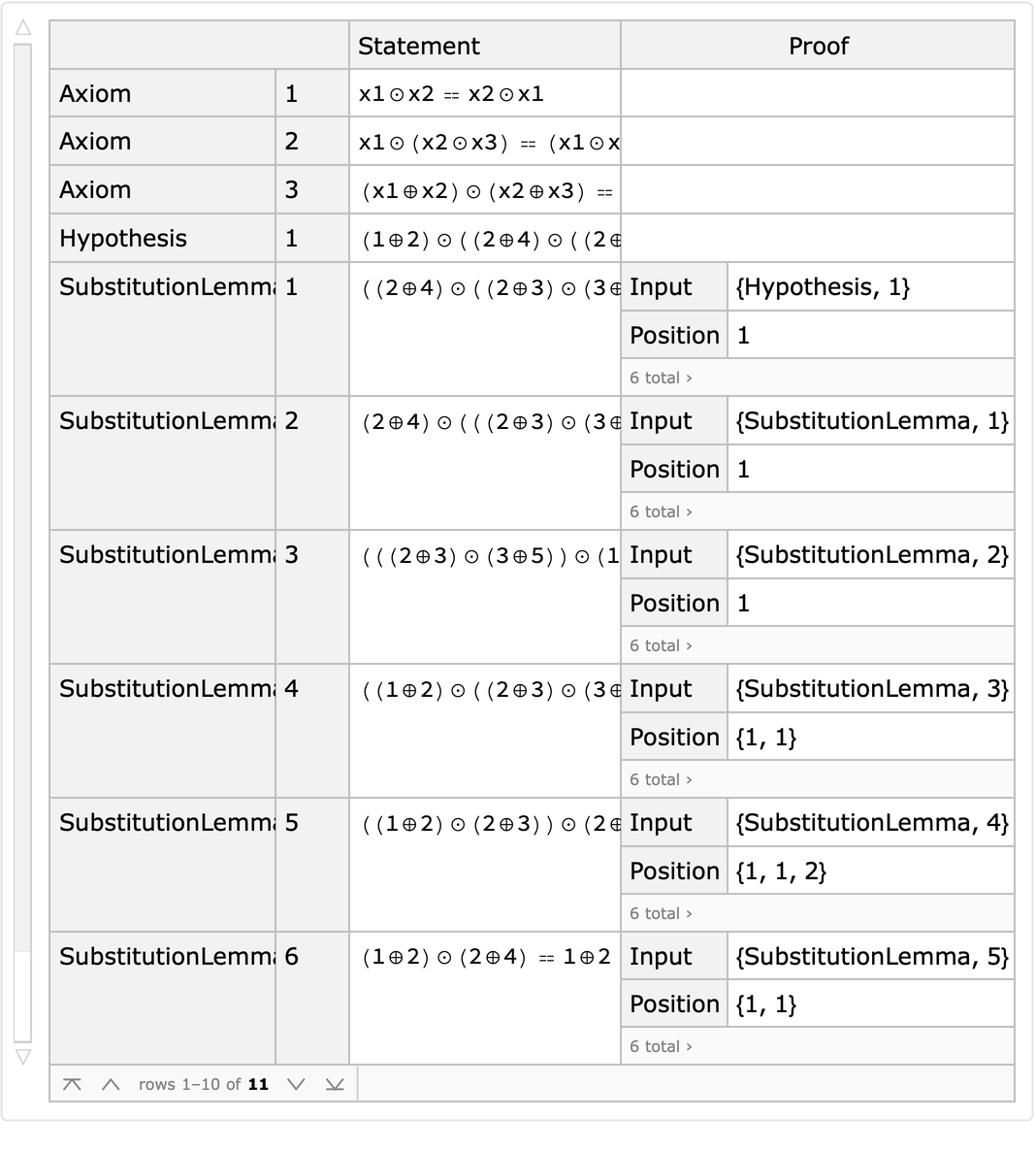Typeset a natural language argument:

 In:=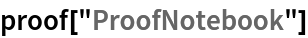Out=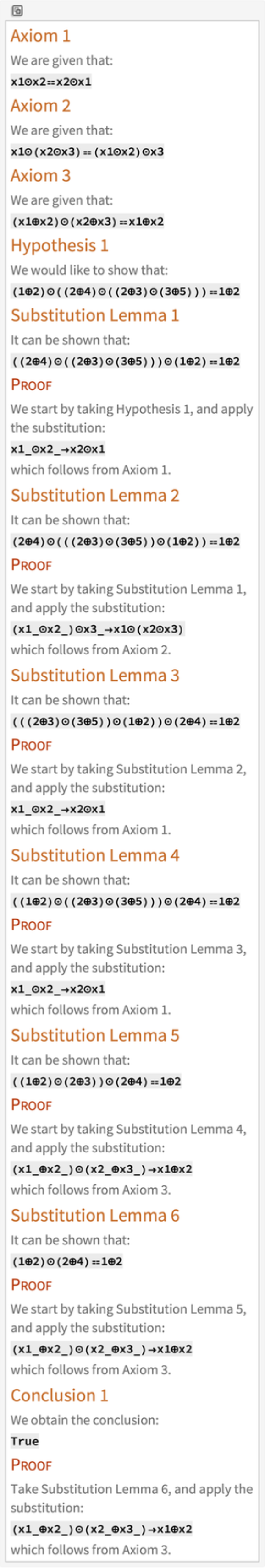Prove a more sophisticated theorem involving multiple rules and hypotheses:

 In:=Out=Show the abstract proof network:

 In:=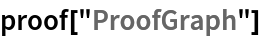Out=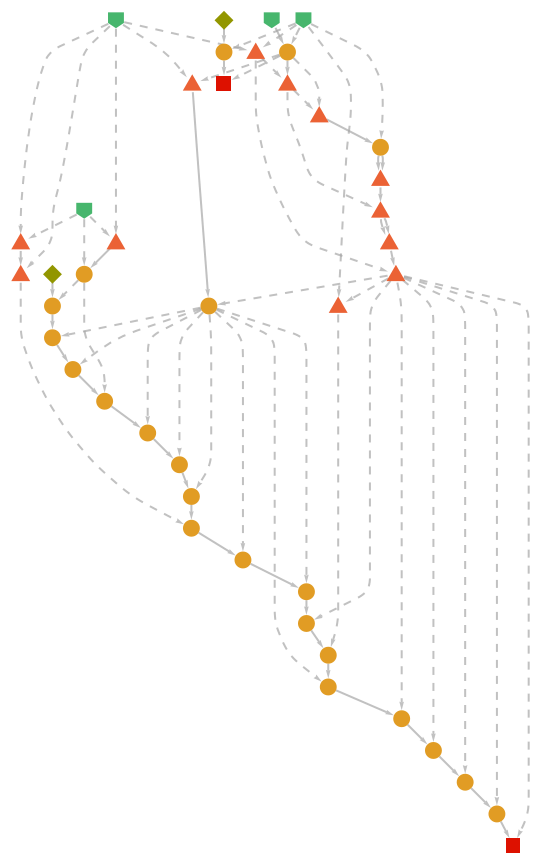Theorems that are true in the case of orderless (undirected) hyperedges may not be true in the case of ordered (directed) ones:

 In:=Out=In:=Out=### Scope (4)

Show that a hypergraph equivalence proposition cannot be derived from a given set of multiway Wolfram model system axioms:

 In:=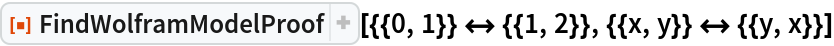Out=#### Rules and Initial Conditions (2)

FindWolframModelProof accepts both individual axioms and lists of axioms:

 In:=Out=In:=Out=Likewise for theorems:

 In:=Out=#### Types of Hyperedges (2)

FindWolframModelProof supports single-vertex edges, ordered two-vertex edges (i.e. ordinary directed edges) and ordered three-vertex hyperedges:

 In:=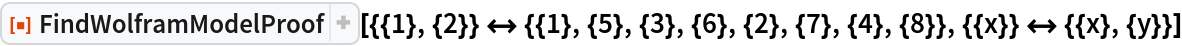Out=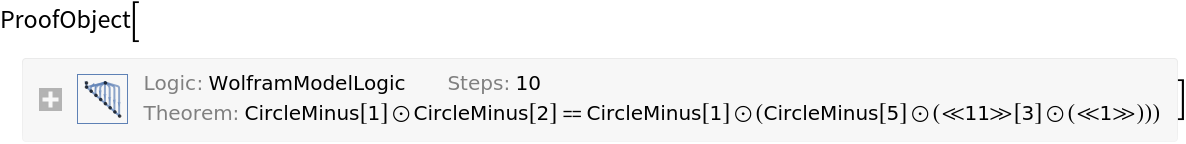In:=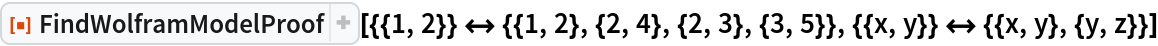Out=In:=Out=As well as combinations of all three:

 In:=Out=### Options (4)

#### TimeConstraint (2)

Use to limit the computation time to t seconds:

 In:=Out=By default, FindWolframModelProof looks for a proof indefinitely:

 In:=Out=#### DirectedHyperedges (2)

By default, all hyperedges are treated as ordered (i.e. directed):

 In:=Out=Use "DirectedHyperedges"False to treat all hyperedges as orderless (i.e. undirected):

 In:=Out=### Properties and Relations (1)

FindWolframModelProof will return a proof object for a particular theorem if and only if the associated path exists in the corresponding multiway system:

 In:=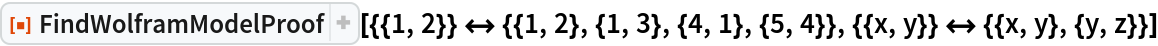Out=In:=Out=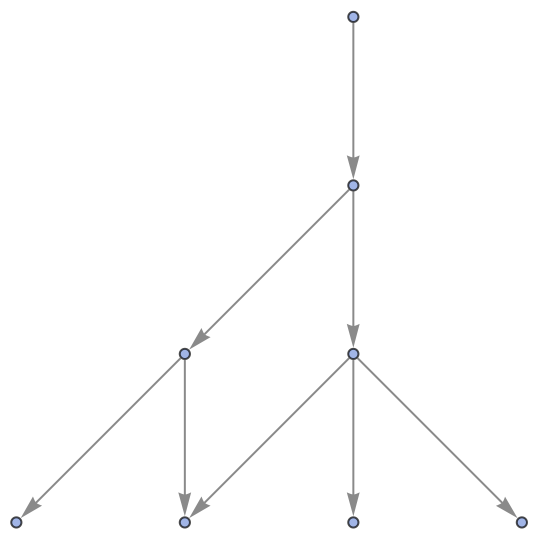In:=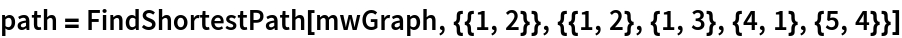Out=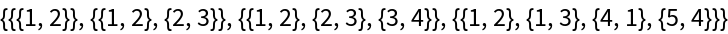In:=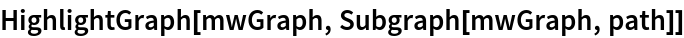Out=